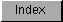```
The IEEE double-precision transcendental math library contains entries for
the transcendental math functions such as sine, cosine, and square root.
The library resides on disk and is opened by calling OpenLibrary() with
"mathieeedoubtrans.library" as the argument. Do not share the library base
pointer between tasks -- see note at beginning of chapter for details.

#include <exec/types.h>
#include <libraries/mathieeedp.h>

#include <clib/mathdoubtrans_protos.h>

struct Library *MathIeeeDoubTransBase;

VOID main()
{
if (MathIeeeDoubTransBase = OpenLibrary("mathieeedoubtrans.library",34))
{
. . .

CloseLibrary(MathIeeeDoubTransBase);
}
else printf("Can't open mathieeedoubtrans.library\n");
}

The global variable MathIeeeDoubTransBase is used internally for all
future library references.

The IEEE double-precision transcendental math library is dependent upon
the IEEE double-precision basic math library, which it will open if it is
not open already.  If you want to use the IEEE double-precision basic math
functions in conjunction with the transcendental math functions however,
you have to specifically open the basic math library yourself.

Just as the IEEE double-precision basic math library, the IEEE
double-precision transcendental math library will take advantage of a
680X0/68881 combination or another math resource, if present.

DP IEEE Transcendental Functions

```